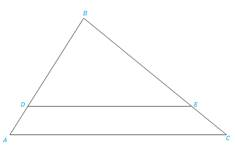Chapter 5.3, Problem 25EElementary Geometry For College St...

7th Edition
Alexander + 2 others
ISBN: 9781337614085

Solutions

Chapter
SectionElementary Geometry For College St...

7th Edition
Alexander + 2 others
ISBN: 9781337614085
Textbook Problem

In Exercises 25 to 28, Δ A B C ∼ Δ D B EExercises 25-28Given AC = 8, DE = 6, CB = 6.Find: EB(HINT: Let EB = x, and solve an equation.)

To determine

To find:

The side EB when the given sides are AC = 8, DE = 6, CB = 6 and ΔABCΔDBE.

Explanation

Definition:

CSSTP:

Corresponding sides of similar triangles are proportional.

Description:

The given figure is shown below.

Figure 1

From the given figure, it is observed that there are two triangles ABC and DBE and which is given that two triangles are similar.

Now draw the two triangles separately as shown below.

Figure 2

Figure 3

Since ΔABCΔDBE and from the definition of CSSTP, corresponding sides of similar triangles are proportional.

That is, BDBA=BEBC=DEAC.

It is given that AC = 8, DE = 6, CB = 6.

Find the side EB.

Consider the last two pairs of proportions BEBC=DEAC in order to find EB

Still sussing out bartleby?

Check out a sample textbook solution.

See a sample solution

The Solution to Your Study Problems

Bartleby provides explanations to thousands of textbook problems written by our experts, many with advanced degrees!

Get Started

A=12bh,b=40,andh=15,findA

Elementary Technical Mathematics

In Exercises 7-12, refer to the following figure. 7. Which point is represented by the ordered pair (4, 2)?

Applied Calculus for the Managerial, Life, and Social Sciences: A Brief Approach

In Problems 33 and 34, find the particular solution to each differential equation.

Mathematical Applications for the Management, Life, and Social Sciences